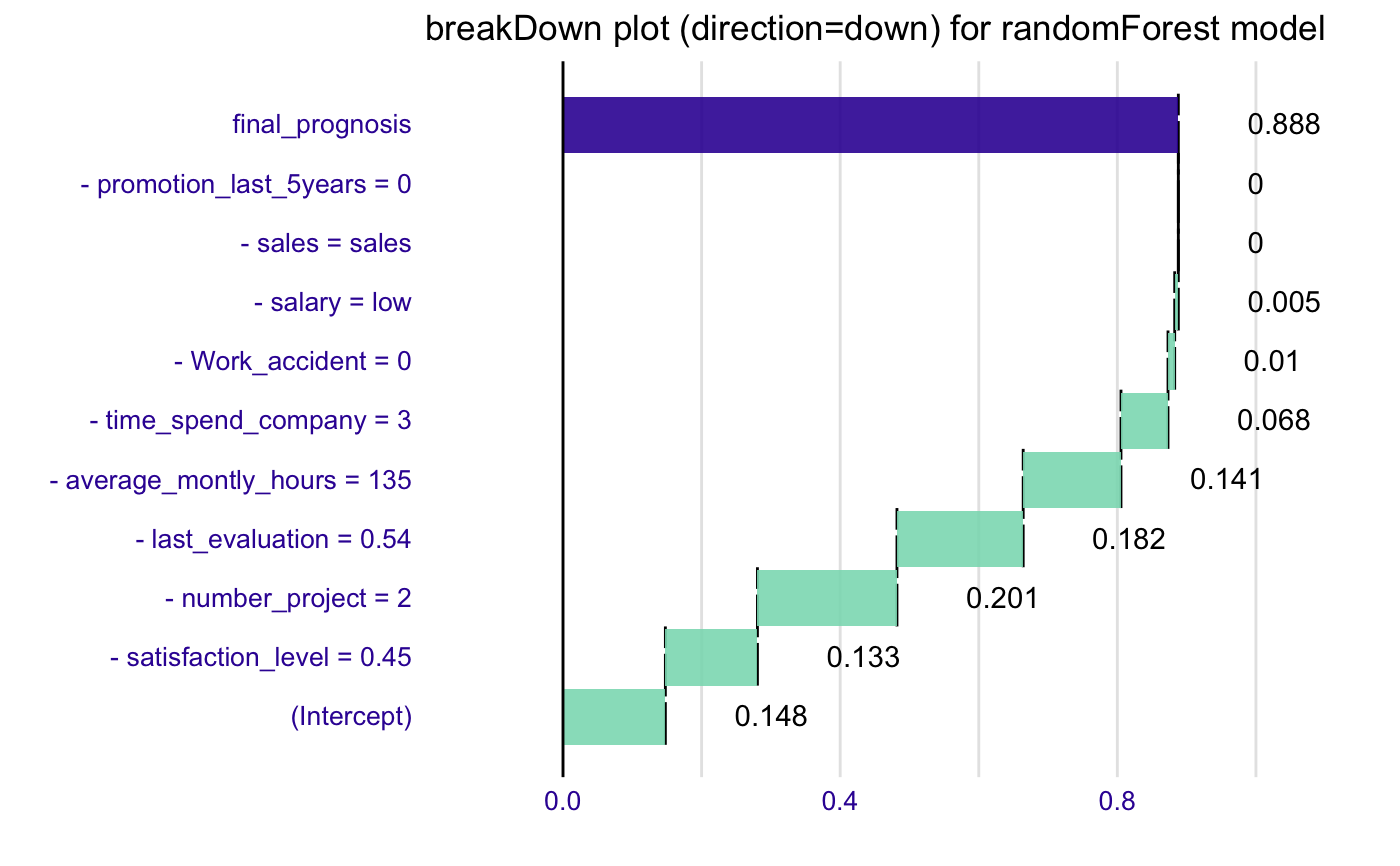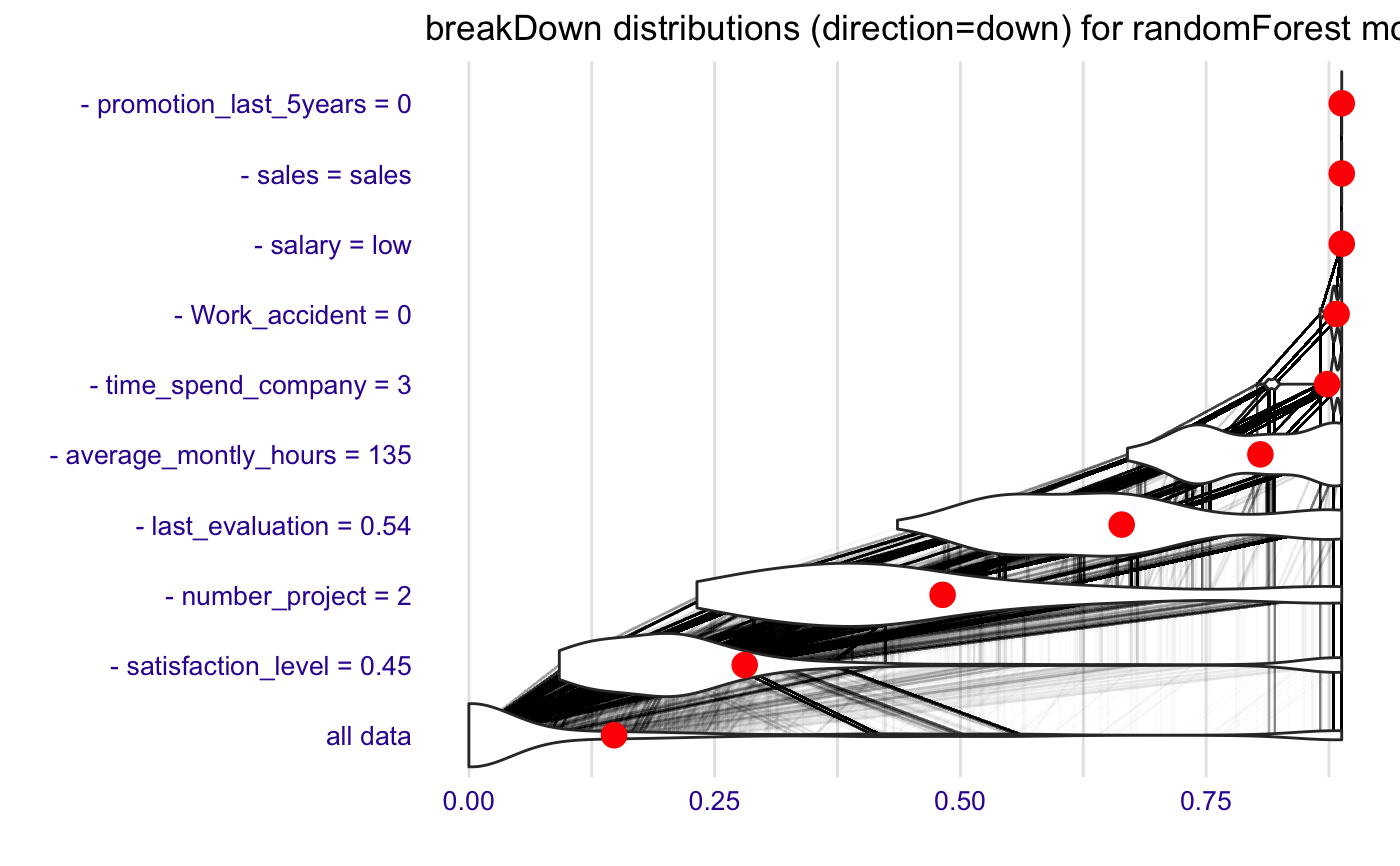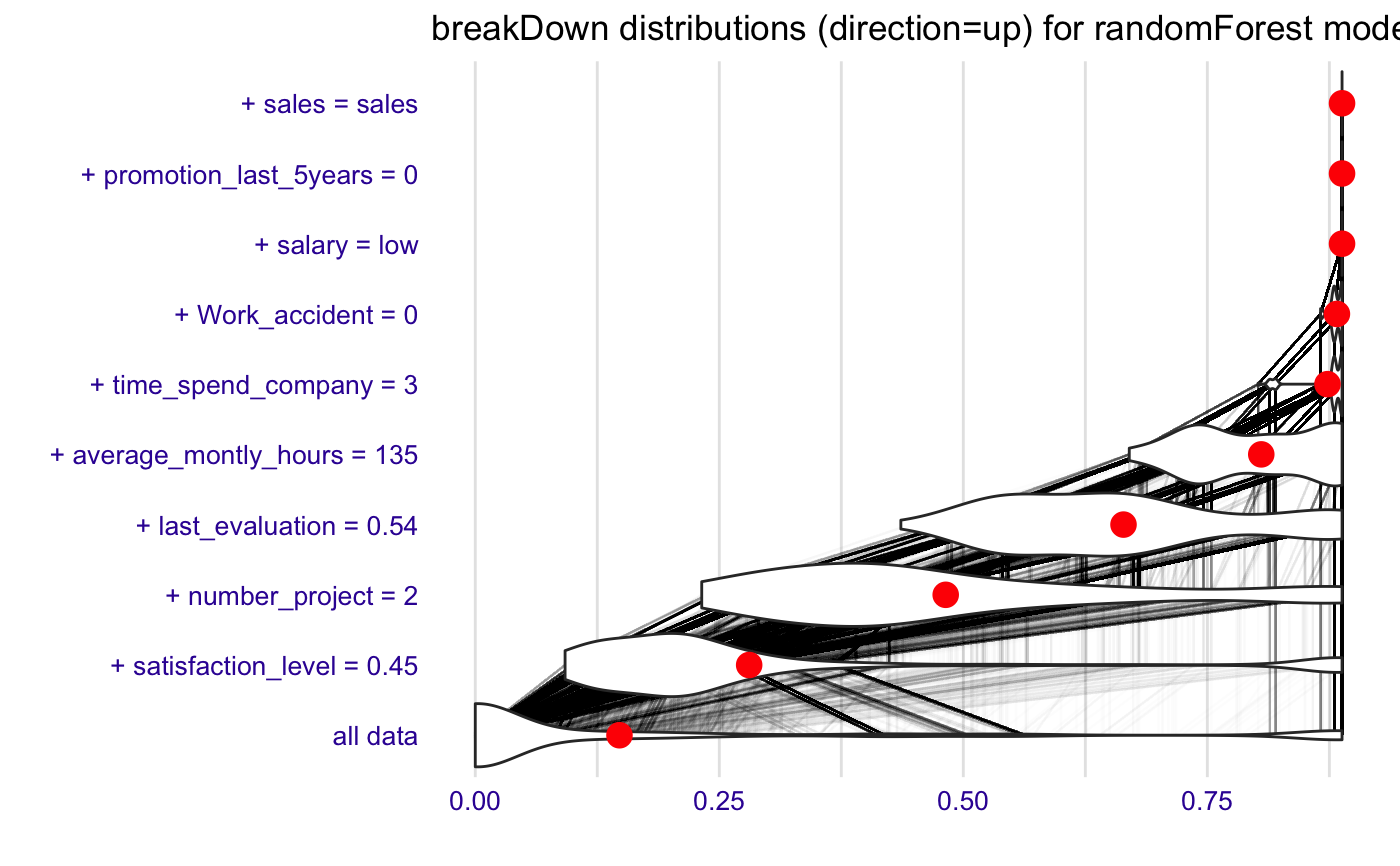The broken function is a generic function for decomposition of model predictions. For linear models please use broken.lm, for generic linear models please use broken.glm. For all other models please use the model agnostic version broken.default. Please note, that some of these functions have additional parameters.

broken(model, new_observation, ...)

## Arguments

model a model a new observation with columns that corresponds to variables used in the model other parameters

## Value

an object of the broken class

## Examples

library("breakDown")
library("randomForest")
library("ggplot2")
set.seed(1313)
model <- randomForest(factor(left)~., data = HR_data, family = "binomial", maxnodes = 5)
predict.function <- function(model, new_observation)
predict(model, new_observation, type="prob")[,2]
predict.function(model, HR_data[11,-7])#>  0.888explain_1 <- broken(model, HR_data[11,-7], data = HR_data[,-7],
predict.function = predict.function, direction = "down")
explain_1#>                              contribution
#> (Intercept)                         0.148
#> - satisfaction_level = 0.45         0.133
#> - number_project = 2                0.201
#> - last_evaluation = 0.54            0.182
#> - average_montly_hours = 135        0.141
#> - time_spend_company = 3            0.068
#> - Work_accident = 0                 0.010
#> - salary = low                      0.005
#> - sales = sales                     0.000
#> - promotion_last_5years = 0         0.000
#> final_prognosis                     0.888
#> baseline:  0 plot(explain_1) + ggtitle("breakDown plot (direction=down) for randomForest model")explain_2 <- broken(model, HR_data[11,-7], data = HR_data[,-7],
predict.function = predict.function, direction = "down", keep_distributions = TRUE)
plot(explain_2, plot_distributions = TRUE) +
ggtitle("breakDown distributions (direction=down) for randomForest model")explain_3 <- broken(model, HR_data[11,-7], data = HR_data[,-7],
predict.function = predict.function, direction = "up", keep_distributions = TRUE)
plot(explain_3, plot_distributions = TRUE) +
ggtitle("breakDown distributions (direction=up) for randomForest model")